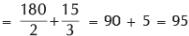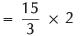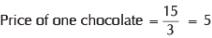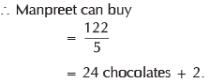Courses

# Olympiad Test: Money - 1

## 10 Questions MCQ Test Mathematics Olympiad Class 4 | Olympiad Test: Money - 1

Description
This mock test of Olympiad Test: Money - 1 for Class 4 helps you for every Class 4 entrance exam. This contains 10 Multiple Choice Questions for Class 4 Olympiad Test: Money - 1 (mcq) to study with solutions a complete question bank. The solved questions answers in this Olympiad Test: Money - 1 quiz give you a good mix of easy questions and tough questions. Class 4 students definitely take this Olympiad Test: Money - 1 exercise for a better result in the exam. You can find other Olympiad Test: Money - 1 extra questions, long questions & short questions for Class 4 on EduRev as well by searching above.
QUESTION: 1

### Golu went to purchase cricket kit and gave to cashier two coins of Rs 5, four notes of Rs 10, three notes of Rs 50 and two notes of Rs 500. If the price of cricket kit was Rs 1162 the change that he would have got back is -------.

Solution:

Required amount
= [2 × 5 + 4 × 10 + 3 × 50
+ 2 × 500] – 1162
= [10 + 40 + 150 + 1000] – 1162
= 1200 = 1162
= Rs 38

QUESTION: 2

### Kapil bought 5 cookies all of which having equal price. If the total amount paid is Rs 33, what was the price of one cookie?

Solution:

Price of one cookie = 33/5 = 6.6
= Rs 6.60

QUESTION: 3

### Direction: Consider the prices of these items below to answer question. Apple --------- Rs 180 per kg Pen --------- Rs 8 per piece Eraser ------- Rs 5 for 2 erasers Chocolates------- Rs 15 for 3 chocolates Q. Ankit wants to buy half kg apples and one chocolate. The total amount he needs to pay is

Solution:

Total amount Ankit needs to payQUESTION: 4

Direction: Consider the prices of these items below to answer question.
Apple --------- Rs 180 per kg
Pen --------- Rs 8 per piece
Eraser ------- Rs 5 for 2 erasers
Chocolates------- Rs 15 for 3 chocolates

Q. If Bunny wants to buy one pen and 3 erasers, how much he needs to pay?

Solution:
QUESTION: 5

Direction: Consider the prices of these items below to answer question.
Apple --------- Rs 180 per kg
Pen --------- Rs 8 per piece
Eraser ------- Rs 5 for 2 erasers
Chocolates------- Rs 15 for 3 chocolates

Q. One kg apples can be bought for Rs 180 and two chocolates can be bought for Rs 7.50 .This statement is ---------.

Solution:

Price of two chocolates= 10
Hence given statement is not correct.

QUESTION: 6

Direction: Consider the prices of these items below to answer question.
Apple --------- Rs 180 per kg
Pen --------- Rs 8 per piece
Eraser ------- Rs 5 for 2 erasers
Chocolates------- Rs 15 for 3 chocolates

Q. If Manpreet has Rs 122 and he wants to buy as many chocolates he can with this amount. The number of chocolates that he can buy is:

Solution:So manpreeet can buy 24 chocolates with 2 rupess still in hand

QUESTION: 7

Direction: Consider the prices of these items below to answer question.
Apple --------- Rs 180 per kg
Pen --------- Rs 8 per piece
Eraser ------- Rs 5 for 2 erasers
Chocolates------- Rs 15 for 3 chocolates
Which of the following statement is false?

Solution:

Eraser cost Rs 5 for 2

so it becomes Rs 2.5 per peice

which means for 6 erasers, we need 6 x 2.5 = Rs 15

which is equivalent to price for 3 chocolates

QUESTION: 8

Yuvraj is very fond of reading books. Once he bought books for Rs 465 and she paid Rs 500 to the bookstore, which expression shows the correct amount of change that he will get back?

Solution:

500 is the amount given and books are for 465
so change given by the shopkeeper is 500-465 Rs

QUESTION: 9

Direction : Consider the following scenario to answer questions.

Shraddha and Shubhra are two friends and one day they decided to go to shopping together. Shraddha had Rs 1500 and Shbuhra had Rs 2000 with them. Shraddha purchase shoes for Rs 550, a skirt for Rs 275 and movie DVD for Rs 50. Shubhra purchased top for Rs 250, a bag for Rs 480, a book for Rs115 and a tennis racket for Rs 500.

Q. What is the total money spent by Shraddha and Shubhra together in shopping?

Solution:

Total amount spent by Shraddha and Shubhra together in shopping
= (550 + 275 + 50) + (250 + 480 + 115 + 500)
= 875 + 1345 = Rs 2220

QUESTION: 10

Direction : Consider the following scenario to answer questions.

Shraddha and Shubhra are two friends and one day they decided to go to shopping together. Shraddha had Rs 1500 and Shbuhra had Rs 2000 with them. Shraddha purchase shoes for Rs 550, a skirt for Rs 275 and movie DVD for Rs 50. Shubhra purchased top for Rs 250, a bag for Rs 480, a book for Rs115 and a tennis racket for Rs 500.

Q. The amount left with Shubhra after shopping is ---------.

Solution:

Amount left with Shubhra after Shopping
= 2000 – 1345 = 655